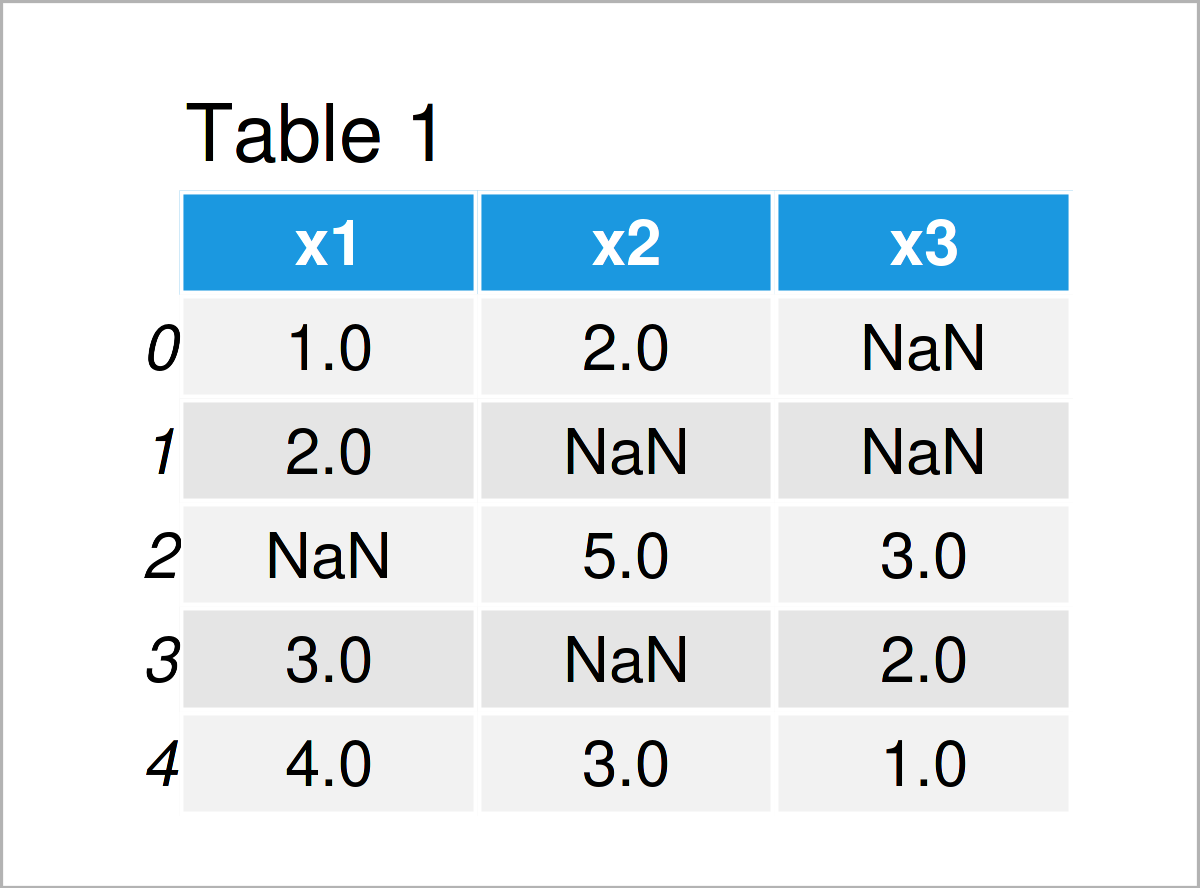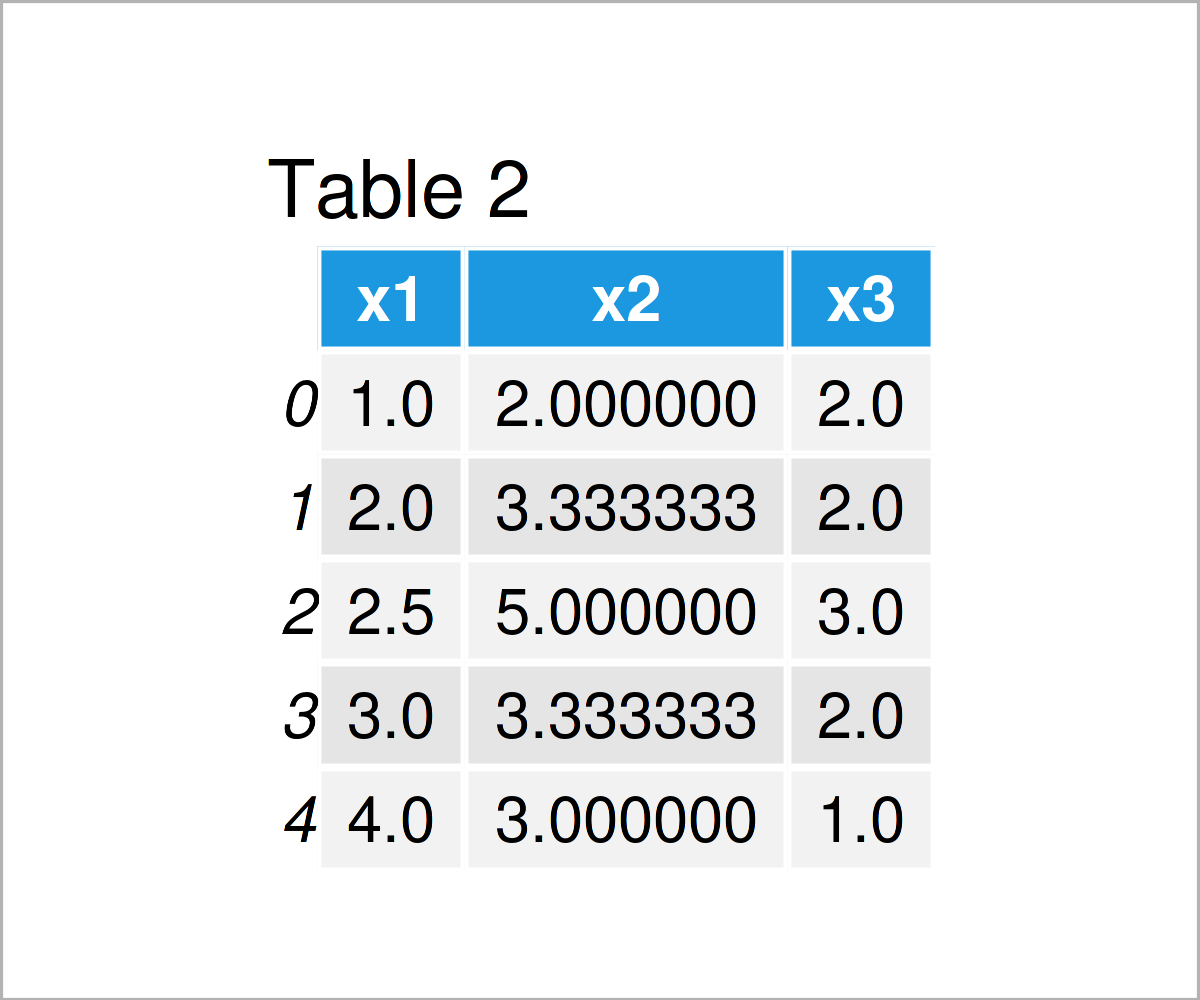# Replace NaN Values by Column Mean in Python (Example)

In this tutorial, I’ll explain how to impute NaN values by the mean of a pandas DataFrame column in the Python programming language.

Let’s just jump right in…

## Example Data & Libraries

First, we need to load the pandas library:

`import pandas as pd                                     # Load pandas library`

In addition, consider the following example data.

```data = pd.DataFrame({'x1':[1, 2, float('NaN'), 3, 4],  # Create example DataFrame
'x2':[2, float('NaN'), 5, float('NaN'), 3],
'x3':[float('NaN'), float('NaN'), 3, 2, 1]})
print(data)                                            # Print example DataFrame```As you can see based on Table 1, our example data is a DataFrame made of five rows and three columns.

All the variables in our data contain at least one missing value.

## Example: Impute Missing Values by Column Mean Using fillna() & mean() Functions

In this example, I’ll explain how to replace NaN values in a pandas DataFrame column by the mean of this column.

Have a look at the following Python code:

```data_new = data.copy()                                 # Create copy of DataFrame
data_new = data_new.fillna(data_new.mean())            # Mean imputation
print(data_new)                                        # Print updated DataFrame```As shown in Table 2, the previous Python syntax has created a new pandas DataFrame where missing values have been exchanged by the mean of the corresponding column.

## Video, Further Resources & Summary

Would you like to know more about the replacing of NaN values by column mean? Then I can recommend having a look at the following video on my YouTube channel. In the video, I show the Python programming code of this article and give some extra explanations:

Please accept YouTube cookies to play this video. By accepting you will be accessing content from YouTube, a service provided by an external third party.If you accept this notice, your choice will be saved and the page will refresh.

In addition, I recommend having a look at the following video on the codebasics YouTube channel. The speaker demonstrates how to handle missing data in a pandas DataFrame in the video:

Please accept YouTube cookies to play this video. By accepting you will be accessing content from YouTube, a service provided by an external third party.If you accept this notice, your choice will be saved and the page will refresh.

Furthermore, you may want to have a look at the other Python tutorials on my homepage. You can find some articles below:

In summary: In this Python tutorial you have learned how to substitute NaN values by the mean of a pandas DataFrame variable. In case you have any further comments and/or questions on missing data imputation by the mean, let me know in the comments.

Subscribe to the Statistics Globe Newsletter